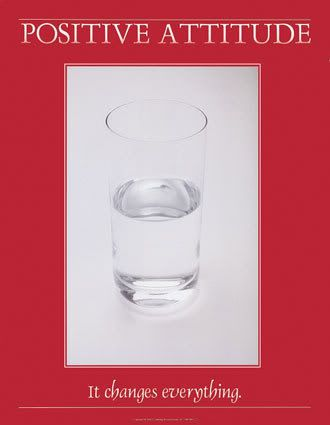## Monday, November 15, 2010

### Learning the Secret : Attitude is EverythingI came across this in a private forum today, and just had to share, I love it.

A small truth to make Life 100% successful....

Let's say: A = 1 B = 2 C = 3 D = 4 E = 5 F = 6 G = 7 H = 8 I = 9 J = 10 K = 11 L = 12 M = 13 N = 14 O = 15 P = 16 Q = 17 R = 18 S = 19 T = 20 U = 21 V = 22 W = 23 X = 24 Y = 25 Z = 26 ______ Then

HARDWORK
H = 8

A = 1

R = 18

D = 4

W = 23

O = 15

R = 18

K = 11

Makes 98% ______

KNOWLEDGE
K = 11

N = 14

O = 15

W = 23

L = 12

E = 5

D = 4

G = 7

E = 5

Makes 96% _____

LOVE

L = 12

O = 15

V = 22

E = 5

Makes 54% _____

LUCK
L = 12

U = 21

C = 3

K = 11

Makes 47%

(None of them makes 100%) _____

Then what makes 100%??

Is it Money? ..... NO!!!

Every problem has a solution, only if we perhaps change our "ATTITUDE".

It is OUR ATTITUDE towards Life and Work that makes OUR Life 100% Successful..

A = 1

T = 20

T = 20

I = 9

T = 20

U = 21

D = 4

E = 5

Makes 100%